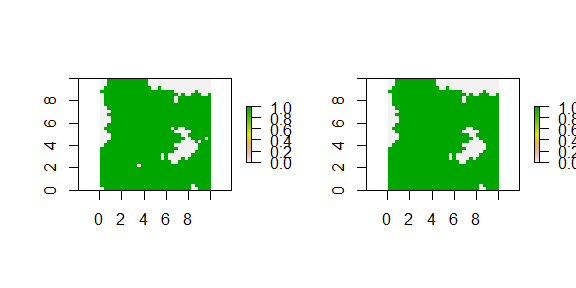# Introduction to ‘landscapeR’

#### 2017-07-05

This document describes the package “landscapeR”. This package is aimed at simulating categorical landscapes on actual geographical realms, starting from either empty landscapes or landscapes provided by the user (e.g. land use maps). The purpose is to provide a tool to tweak or create the landscape while retaining a high degree of control on its features, without the hassle of specifying each location attribute. In this it differs from other tools which generate null or neutral landscape in a theorethical space. Input and outputs are raster datasets or R integer vectors indicating the raster cell indexes. Cell indexes always range from 1 to the number of cells composing the raster including missing values (i.e. the number of columns times number of rows) and are assigned from left to right and top to bottom. In landscapeR, areas are measured by number of raster cells, rather than actual areas. Therefore, areas (and dimensions) of cells within a raster are always treated as equal, as the package is conceived to work on landscapes from fine to mid scale, where differences in cell areas due to latitude are relatively small. This may be an issue when working with non projected data at very wide scale (e.g. from subcontinental to global). All basic GIS operations are handled by the raster package.

Below it follows a set of examples, using landscapeR functions to generate various landscape configurations. Let’s start loading the required packages and making an empty landscape (by transforming a matrix into a raster a geographical object of class RasterLayer):

library(landscapeR)
library(raster)
m <- matrix(0, 33, 33)
r <- raster(m, xmn=0, xmx=10, ymn=0, ymx=10)

### makePatch

This is the basic function to create a single patch. However, makeClass should be preferred, even when creating a single patch (see below). By default, makePatch returns a vector of cell indexes, so to plot a map argument rast=TRUE. More features can be specified about the patch, but makeClass provide better exception/error handling.

rr <- makePatch(r, size=500, rast=TRUE)
plot(rr)

### makeClass

makeClass generates a group of patches, as specified by arguments. Example:

num <- 5
size <- 15
rr <- makeClass(r, num, size)
plot(rr)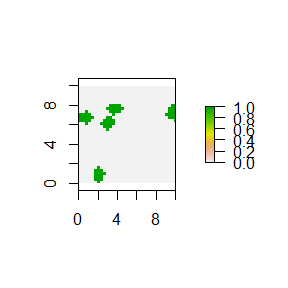Patches are allowed to be contiguous, so they may appear as a single patch in those instances:

num <- 75
size <- 10
rr <- makeClass(r, num, size)
plot(rr)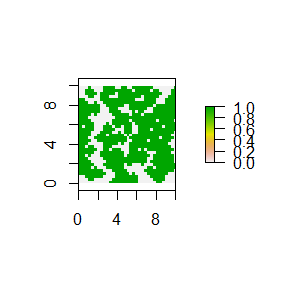Each patch size and seed starting position (by cell index, left to right, top to bottom) can be specified as well:

num <- 5
size <- c(1,5,10,20,50)
pts <- c(1, 33, 1089, 1057, 545)
rr <- makeClass(r, num, size, pts)
plot(rr)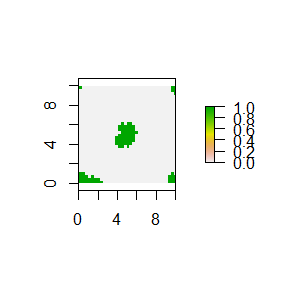Background (argument bgr) can be one or more classes:

rr <- makeClass(r, 3, 100, val=1)
rr <- makeClass(rr, 5, 50, val=3) ## Creates a second class in the landscape with value 3
par(mfrow=c(1,2))
plot(rr)
rr <- makeClass(rr, 1, 250, bgr=c(0,1), val=2) ## Builds a third class, allowed on background and class 1.
plot(rr)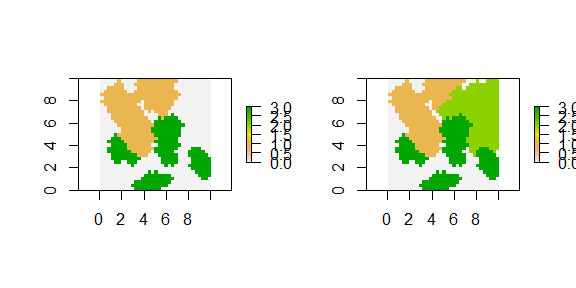makeClass should be used preferably when creating a single patch, as better error and exception handling is provided. To create a single patch:

rr <- makeClass(r, 1, size=500)
plot(rr)

Some more features can be specified about the patch. For example, the following will create a patch with value 2, starting from the centre cell of the raster:

patchSize <- 500
class <- 2
centre <- 545
rr <- makeClass(r, 1, patchSize, centre, val=class)
plot(rr)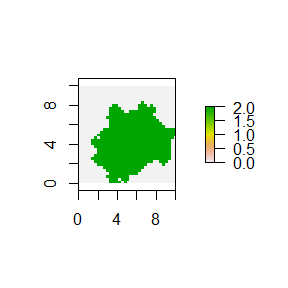Forbidden cells can be specified by value, so the patch will occupy only the allowed background. The following will generate a new patch with value 1 and size 100 inside the patch created previously:

rr <- makeClass(rr, 1, 100, bgr=class, val=1)
plot(rr, axes=FALSE)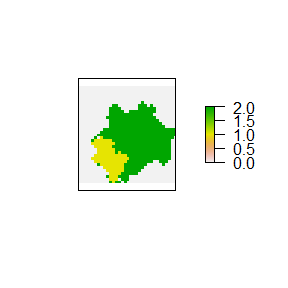### expandClass

Expands (and shrinks) classes starting from an existing landscape. Below, in the right plot, class 1 is expanded by 250 cells:

rr <- makeClass(r, 1, patchSize, centre)
par(mfrow=c(1,2))
plot(rr)
rex <- expandClass(rr, 1, size=250)
plot(rex)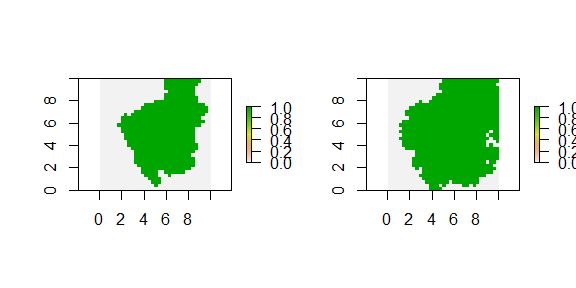Multiple background values are allowed, when the class has to expand over two or more existing classes:

rr <- makeClass(r, 5, 100)
rr <- makeClass(rr, 5, 50, val=2) ## Creates a second class in the landscape with value 2
par(mfrow=c(1,2))
plot(rr)
rex <- expandClass(rr, 2, 250, bgr = c(0,1))
plot(rex)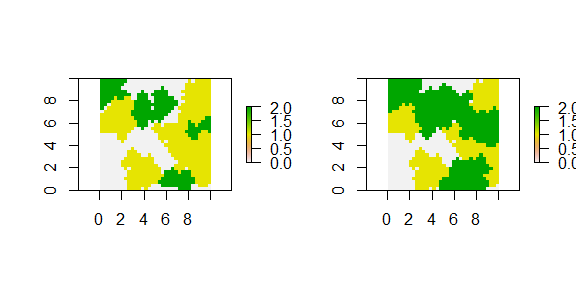This function can be used to mimic shapes, by providing a skeleton:

m[,17] <- 1
r <- raster(m, xmn=0, xmx=10, ymn=0, ymx=10)
par(mfrow=c(1,2))
plot(r)
rr <- expandClass(r, 1, 200)
plot(rr)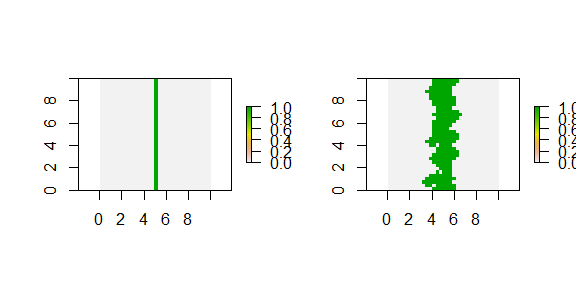### makeLine

Creates a linear feature, at a given convolution level and direction in degrees (zero is North)

m[] <- 0
r <- raster(m, xmn=0, xmx=10, ymn=0, ymx=10)
rr <- makeLine(r, size=50, spt = 545, direction=45, convol=0.05, val=2, rast=TRUE)
plot(rr)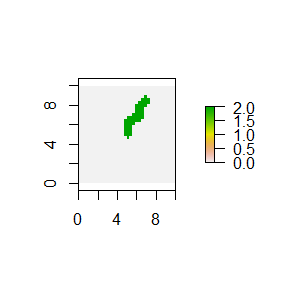In combination with expandClass anisotropic features or landscapes can be created:

rr <- matrix(0,100,100)
rr <- raster(rr, xmn=0, xmx=10, ymn=0, ymx=10)
for(i in c(550, 3050, 5050, 7550)){
rr = makeLine(rr, size=50, rast=TRUE, spt=i, direction=135, convol=0.25)
}
plot(expandClass(rr, 1, 250))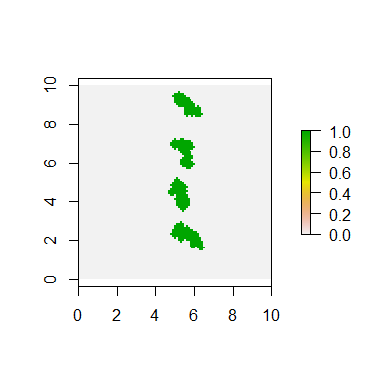### rmSingle

Removes single tones from patches, reducing salt-pepper effect. The cells values are assigned from one random neighbouring cell (right plot).

rr <- makeClass(r, 10, 100)
par(mfrow=c(1,2))
plot(rr)
rs <- rmSingle(rr)
plot(rs)